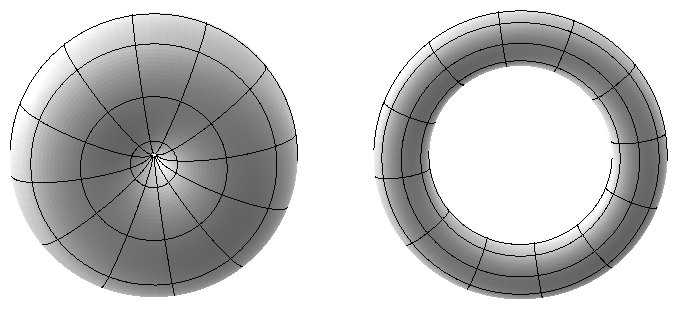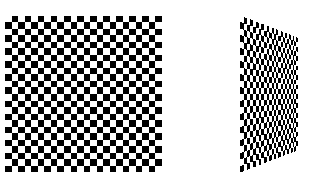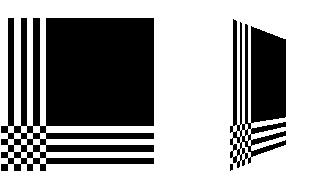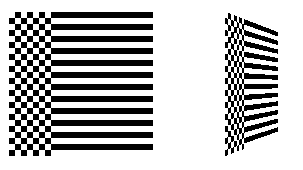## Assigning Texture Coordinates

As you draw your texture-mapped scene, you must provide both object coordinates and texture coordinates for each vertex. After transformation, the object coordinates determine where on the screen that particular vertex is rendered. The texture coordinates determine which texel in the texture map is assigned to that vertex. In exactly the same way that colors are interpolated between two vertices of shaded polygons and lines, texture coordinates are also interpolated between vertices. (Remember that textures are rectangular arrays of data.)

Texture coordinates can comprise one, two, three, or four coordinates. They're usually referred to as the s, t, r, and q coordinates to distinguish them from object coordinates (x, y, z, and w) and from evaluator coordinates (u and v; see Chapter 12). For one-dimensional textures, you use the s coordinate; for two-dimensional textures, you use s and t. In Release 1.1, the r coordinate is ignored. (Some implementations have 3D texture mapping as an extension, and that extension uses the r coordinate.) The q coordinate, like w, is typically given the value 1 and can be used to create homogeneous coordinates; it's described as an advanced feature in "The q Coordinate." The command to specify texture coordinates, glTexCoord*(), is similar to glVertex*(), glColor*(), and glNormal*() - it comes in similar variations and is used the same way between glBegin() and glEnd() pairs. Usually, texture-coordinate values range from 0 to 1; values can be assigned outside this range, however, with the results described in "Repeating and Clamping Textures."

void glTexCoord{1234}{sifd}(TYPEcoords);
void glTexCoord{1234}{sifd}v(TYPE *coords);

Sets the current texture coordinates (s, t, r, q). Subsequent calls to glVertex*() result in those vertices being assigned the current texture coordinates. With glTexCoord1*(), the s coordinate is set to the specified value, t and r are set to 0, and q is set to 1. Using glTexCoord2*() allows you to specify s and t; r and q are set to 0 and 1, respectively. With glTexCoord3*(), q is set to 1 and the other coordinates are set as specified. You can specify all coordinates with glTexCoord4*(). Use the appropriate suffix (s, i, f, or d) and the corresponding value for TYPE (GLshort, GLint, GLfloat, or GLdouble) to specify the coordinates' data type. You can supply the coordinates individually, or you can use the vector version of the command to supply them in a single array. Texture coordinates are multiplied by the 4 × 4 texture matrix before any texture mapping occurs. (See "The Texture Matrix Stack.") Note that integer texture coordinates are interpreted directly rather than being mapped to the range [-1,1] as normal coordinates are.

The next section discusses how to calculate appropriate texture coordinates. Instead of explicitly assigning them yourself, you can choose to have texture coordinates calculated automatically by OpenGL as a function of the vertex coordinates. (See "Automatic Texture-Coordinate Generation.")

### Computing Appropriate Texture Coordinates

Two-dimensional textures are square or rectangular images that are typically mapped to the polygons that make up a polygonal model. In the simplest case, you're mapping a rectangular texture onto a model that's also rectangular - for example, your texture is a scanned image of a brick wall, and your rectangle is to represent a brick wall of a building. Suppose the brick wall is square and the texture is square, and you want to map the whole texture to the whole wall. The texture coordinates of the texture square are (0, 0), (1, 0), (1, 1), and (0, 1) in counterclockwise order. When you're drawing the wall, just give those four coordinate sets as the texture coordinates as you specify the wall's vertices in counterclockwise order.

Now suppose that the wall is two-thirds as high as it is wide, and that the texture is again square. To avoid distorting the texture, you need to map the wall to a portion of the texture map so that the aspect ratio of the texture is preserved. Suppose that you decide to use the lower two-thirds of the texture map to texture the wall. In this case, use texture coordinates of (0,0), (1,0), (1,2/3), and (0,2/3) for the texture coordinates as the wall vertices are traversed in a counterclockwise order.

As a slightly more complicated example, suppose you'd like to display a tin can with a label wrapped around it on the screen. To obtain the texture, you purchase a can, remove the label, and scan it in. Suppose the label is 4 units tall and 12 units around, which yields an aspect ratio of 3 to 1. Since textures must have aspect ratios of 2n to 1, you can either simply not use the top third of the texture, or you can cut and paste the texture until it has the necessary aspect ratio. Suppose you decide not to use the top third. Now suppose the tin can is a cylinder approximated by thirty polygons of length 4 units (the height of the can) and width 12/30 (1/30 of the circumference of the can). You can use the following texture coordinates for each of the thirty approximating rectangles:

1: (0, 0), (1/30, 0), (1/30, 2/3), (0, 2/3)

2: (1/30, 0), (2/30, 0), (2/30, 2/3), (1/30, 2/3)

3: (2/30, 0), (3/30, 0), (3/30, 2/3), (2/30, 2/3)

. . .

30: (29/30, 0), (1, 0), (1, 2/3), (29/30, 2/3)

Only a few curved surfaces such as cones and cylinders can be mapped to a flat surface without geodesic distortion. Any other shape requires some distortion. In general, the higher the curvature of the surface, the more distortion of the texture is required.

If you don't care about texture distortion, it's often quite easy to find a reasonable mapping. For example, consider a sphere whose surface coordinates are given by (cos &thgr; cos &phgr; , cos &thgr; sin &phgr; , sin &thgr; ), where 0 ≤ &thgr; ≤ 2 &pgr; , and 0 ≤ &phgr; ≤ &pgr; . The &thgr; - &phgr; rectangle can be mapped directly to a rectangular texture map, but the closer you get to the poles, the more distorted the texture is. The entire top edge of the texture map is mapped to the north pole, and the entire bottom edge to the south pole. For other surfaces, such as that of a torus (doughnut) with a large hole, the natural surface coordinates map to the texture coordinates in a way that produces only a little distortion, so it might be suitable for many applications. Figure 9-6 shows two tori, one with a small hole (and therefore a lot of distortion near the center) and one with a large hole (and only a little distortion).Figure 9-6 : Texture-Map Distortion

If you're texturing spline surfaces generated with evaluators (see Chapter 12), the u and v parameters for the surface can sometimes be used as texture coordinates. In general, however, there's a large artistic component to successfully mapping textures to polygonal approximations of curved surfaces.

### Repeating and Clamping Textures

You can assign texture coordinates outside the range [0,1] and have them either clamp or repeat in the texture map. With repeating textures, if you have a large plane with texture coordinates running from 0.0 to 10.0 in both directions, for example, you'll get 100 copies of the texture tiled together on the screen. During repeating, the integer part of texture coordinates is ignored, and copies of the texture map tile the surface. For most applications where the texture is to be repeated, the texels at the top of the texture should match those at the bottom, and similarly for the left and right edges.

The other possibility is to clamp the texture coordinates: Any values greater than 1.0 are set to 1.0, and any values less than 0.0 are set to 0.0. Clamping is useful for applications where you want a single copy of the texture to appear on a large surface. If the surface-texture coordinates range from 0.0 to 10.0 in both directions, one copy of the texture appears in the lower corner of the surface. If you've chosen GL_LINEAR as the filtering method (see "Filtering"), an equally weighted combination of the border color and the texture color is used, as follows.

• When repeating, the 2 × 2 array wraps to the opposite edge of the texture. Thus, texels on the right edge are averaged with those on the left, and top and bottom texels are also averaged.
• If there is a border, then the texel from the border is used in the weighting. Otherwise, GL_TEXTURE_BORDER_COLOR is used. (If you've chosen GL_NEAREST as the filtering method, the border color is completely ignored.)

Note that if you are using clamping, you can avoid having the rest of the surface affected by the texture. To do this, use alpha values of 0 for the edges (or borders, if they are specified) of the texture. The decal texture function directly uses the texture's alpha value in its calculations. If you are using one of the other texture functions, you may also need to enable blending with good source and destination factors. (See "Blending" in Chapter 6.)

To see the effects of wrapping, you must have texture coordinates that venture beyond [0.0, 1.0]. Start with Example 9-1, and modify the texture coordinates for the squares by mapping the texture coordinates from 0.0 to 3.0 as follows:

`glBegin(GL_QUADS);`
`  glTexCoord2f(0.0, 0.0); glVertex3f(-2.0, -1.0, 0.0);     `
`  glTexCoord2f(0.0, 3.0); glVertex3f(-2.0, 1.0, 0.0);`
`  glTexCoord2f(3.0, 3.0); glVertex3f(0.0, 1.0, 0.0);`
`  glTexCoord2f(3.0, 0.0); glVertex3f(0.0, -1.0, 0.0);`
` `
`  glTexCoord2f(0.0, 0.0); glVertex3f(1.0, -1.0, 0.0);`
`  glTexCoord2f(0.0, 3.0); glVertex3f(1.0, 1.0, 0.0); `
`  glTexCoord2f(3.0, 3.0); glVertex3f(2.41421, 1.0, -1.41421);`
`  glTexCoord2f(3.0, 0.0); glVertex3f(2.41421, -1.0, -1.41421); glEnd();`

With GL_REPEAT wrapping, the result is as shown in Figure 9-7.Figure 9-7 : Repeating a Texture

In this case, the texture is repeated in both the s and t directions, since the following calls are made to glTexParameter*():

`glTexParameteri(GL_TEXTURE_2D, GL_TEXTURE_WRAP_S, GL_REPEAT);`
`glTexParameteri(GL_TEXTURE_2D, GL_TEXTURE_WRAP_T, GL_REPEAT);`

If GL_CLAMP is used instead of GL_REPEAT for each direction, you see something similar to Figure 9-8.Figure 9-8 : Clamping a Texture

You can also clamp in one direction and repeat in the other, as shown in Figure 9-9.Figure 9-9 : Repeating and Clamping a Texture

You've now seen all the possible arguments for glTexParameter*(), which is summarized here.

void glTexParameter{if}(GLenum target, GLenum pname, TYPE param);
void glTexParameter{if}v(GLenum target, GLenum pname,
TYPE *param);

Sets various parameters that control how a texture is treated as it's applied to a fragment or stored in a texture object. The target parameter is either GL_TEXTURE_2D or GL_TEXTURE_1D to indicate a two- or one-dimensional texture. The possible values for pname and param are shown in Table 9-4. You can use the vector version of the command to supply an array of values for GL_TEXTURE_BORDER_COLOR, or you can supply individual values for other parameters using the nonvector version. If these values are supplied as integers, they're converted to floating-point according to Table 4-1; they're also clamped to the range [0,1].

 Table 9-4 : glTexParameter*() Parameters Parameter Values GL_TEXTURE_WRAP_S GL_CLAMP, GL_REPEAT GL_TEXTURE_WRAP_T GL_CLAMP, GL_REPEAT GL_TEXTURE_MAG_FILTER GL_NEAREST, GL_LINEAR GL_TEXTURE_MIN_FILTER GL_NEAREST, GL_LINEAR, GL_NEAREST_MIPMAP_NEAREST, GL_NEAREST_MIPMAP_LINEAR, GL_LINEAR_MIPMAP_NEAREST, GL_LINEAR_MIPMAP_LINEAR GL_TEXTURE_BORDER_COLOR any four values in [0.0, 1.0] GL_TEXTURE_PRIORITY [0.0, 1.0] for the current texture object

Try This

Figure 9-8 and Figure 9-9 are drawn using GL_NEAREST for the minification and magnification filter. What happens if you change the filter values to GL_LINEAR? Why?